Next: FFTCN: Multi-Dimensional Complex Fourier Up: How FFTEASY works Previous: How FFTEASY works

## FFTC1: One-Dimensional Complex Fourier Transforms

Whether you prove them to yourself or take my word for them the formulas given above should be enough for you to follow what the routine fftc1 does. The first section takes an array ofpoints and rearranges them into bit-reversed order. This is done by noting that if you know the bit-reversalof a binary number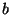it is easy to find the bit-reversal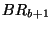of the number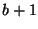. To addtoyou start at the right and replace everywith a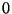until you reach the first, which you replace with a. So to go fromtoyou do the bit-reverse of this process, starting at the left and replacing everywith auntil you reach the first, which you replace with a.

The next part of the routine implements the DL formula on successively larger blocks, beginning with combiningtransforms into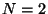transforms. Each time the routine calculates a transform of sizethe first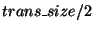elements represent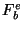and the next half represent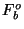. These are then used to calculateand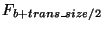. Note that in the DL formula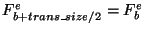,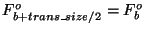, and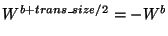, so the only difference between the formulas forandis the sign of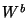. Finally, the inner loop over the variableiterates over all the transforms of sizein the entire array, so if you are transforming anarray of data you first have to calculate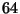transforms, thentransforms, and so on.

Note that the formulas for the real and imaginary parts of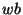are nothing butand. They could have simply been calculated directly but for the sake of efficiency they are calculated instead using a recursion formula each timeis incremented. This formula can be verified simply using the addition rules for sines and cosines.

Note that the end result of this process is a transform with the results in sequential order fromto, which by the periodicity ofis exactly the storage scheme described in section 2.3.2.

The reverse transform is exactly identical except the sign of the exponential is reversed and the final output is divided by.

The argument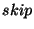is used in case the elements of the input array are not sequential but evenly spaced in some larger array. To do a one-dimensional transform you would almost always want to setto one, but this option allows fftc1 to be used by fftcn, which does transforms of multi-dimensional arrays. For all but the last array index the one-dimensional transforms used by fftcn are not of sequential data.Next: FFTCN: Multi-Dimensional Complex Fourier Up: How FFTEASY works Previous: How FFTEASY works